## Number System Conversions-

Before you go through this article, make sure that you have gone through the previous article on Basics of Number System.

In number system,

• It is very important to have a good knowledge of how to convert numbers from one base to another base.
• Here, we will learn how to convert any given number from any base to any other base.## Conversion of Bases-

A given number in base x can be converted to any other base y using the following steps-

### Step-01:

Convert the number from base x to base 10 using expansion method.

Read More- Conversion to Base 10

### Step-02:

Convert the number from base 10 to base y using division & multiplication method.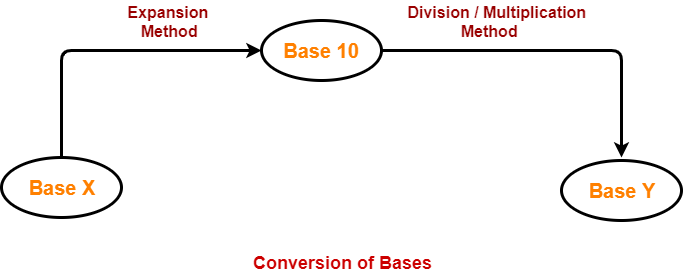Read More-

## Problem-01:

Convert (1056)16 to ( ? )8

## Solution-

### (1056)16 → ( ? )10

Using Expansion method, we have-

(1056)16

= 1 x 163 + 0 x 162 + 5 x 161 + 6 x 160

= 4096 + 0 + 80 + 6

= (4182)10

From here, (1056)16 = (4182)10

### (4182)10 → ( ? )8

Using Division method, we have-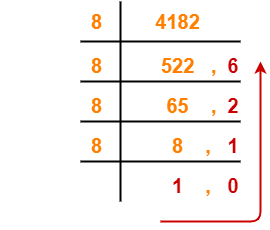From here, (4182)10 = (10126)8

Thus, (1056)16 = (10126)8

## Problem-02:

Convert (11672)8 to ( ? )16

## Solution-

### (11672)8 → ( ? )10

Using Expansion method, we have-

(11672)8

= 1 x 84 + 1 x 83 + 6 x 82 + 7 x 81 + 2 x 80

= 4096 + 512 + 384 + 56 + 2

= (5050)10

From here, (11672)8 = (5050)10

### (5050)10 → ( ? )16

Using Division method, we have-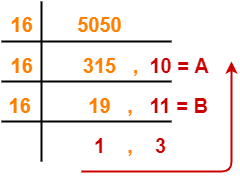From here, (5050)10 = (13BA)16

Thus, (11672)8 = (13BA)16

## Problem-03:

Convert (2724)8 to ( ? )5

## Solution-

### (2724)8 → ( ? )10

Using Expansion method, we have-

(2724)8

= 2 x 83 + 7 x 82 + 2 x 81 + 4 x 80

= 1024 + 448 + 16 + 4

= (1492)10

From here, (2724)8 = (1492)10

### (1492)10 → ( ? )5

Using Division method, we have-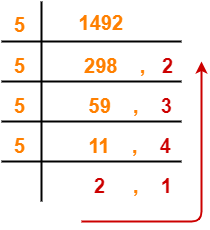From here, (1492)10 = (21432)5

Thus, (2724)8 = (21432)5

## Problem-04:

Convert (3211)4 to ( ? )5

## Solution-

### (3211)4 → ( ? )10

Using Expansion method, we have-

(3211)4

= 3 x 43 + 2 x 42 + 1 x 41 + 1 x 40

= 192 + 32 + 4 + 1

= (229)10

From here, (3211)4 = (229)10

### (229)10 → ( ? )5

Using Division method, we have-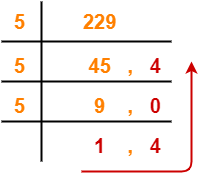From here, (229)10 = (1404)5

Thus, (3211)4 = (1404)5

## Problem-05:

Convert (1001001100)2 to ( ? )6

## Solution-

### (1001001100)2 → ( ? )10

Using Expansion method, we have-

(1001001100)2

= 1 x 29 + 0 x 28 + 0 x 27 + 1 x 26 + 0 x 25 + 0 x 24 + 1 x 23 + 1 x 22 + 0 x 21 + 0 x 20

= 512 + 64 + 8 + 4

= (588)10

From here, (1001001100)2 = (588)10

### (588)10 → ( ? )6

Using Division method, we have-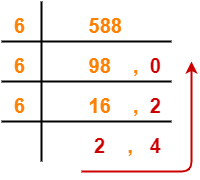From here, (588)10 = (2420)6

Thus, (1001001100)2 = (2420)6

To gain better understanding about Conversion of Bases,

Watch this Video Lecture

Get more notes and other study material of Number System.

Watch video lectures by visiting our YouTube channel LearnVidFun.